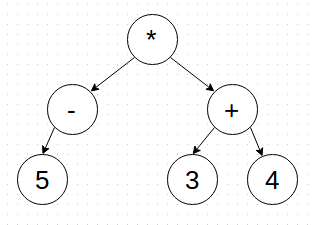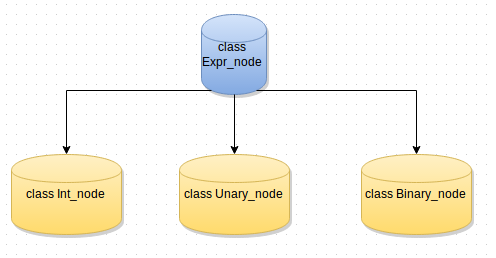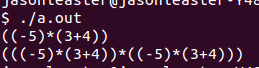Generally, OOP( Object Oriented Progarmming) have three important elements:

• Abstraction of data
• Inheritance
• Dynamic Binding

Now, there is a demo for how to build an arithmetic expression-tree.We want to build a node-tree like the tree in that picture to print a correct arithmetic expression.

Here you could see the relationship between the classes we used in our demo.You know that the three classes `Int_node`, `Unary_node` and `Binary_node` are all inherit from the base class `Expr_node`.

Let’s look at the simple unary arithmetic expression `(-5)`. In this expression, there is only one operand `-` and one integer `5`. In expression `( 3 + 4)`, there are two integer and one operand.

We could find that there are only three different expression in representation of arithmetic expression. They are:

• Integer Expression
• Unary Expression
• Binary Expression

So, we find the common attributes on the three different types of expression and use a base-class `Expr_node` to represent this attribute.

If you have background in class handle, you may notice that `class Expr` is the handle for `Expr_node`.

We don’t need real objects of `class Expr_node`. What we need is the classes which inherit from that base-class. The meaning of the base-class is to provide the public interface.

You could run this program and will get the output like this one.### More Opeartion

We could evaluate the expression and add more types of node.

Here is the implementation. That’s cool!Photo by Zhouyin in ShangHai, China